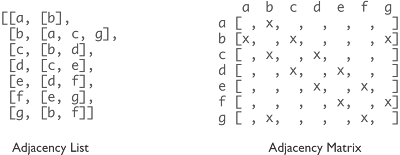## Monday, 3 December 2012

### The Right Representation for the Job

All programmers know that premature-optimisation is the root of all evil. Sometimes however, you just can't shake the hunch that something really is slowing your code down. It's often wise to try to suppress such thoughts as in reality it probably won't make much difference. Occasionally it does actual matter.

This particular case concerns the representation of (chemical) graphs. Below shows a simple undirected graph with seven vertexes, seven edges and a single cycle.simple graph

We can store this in several ways. Wikipedia has a nice article on the abstract data type which also lists the time complexity of operations on each representation.Representations of the simple graph. The adjacency list, lists all adjacent vertices for each vertex. The adjacency matrix indicates which  vertices are connected by marking the appropriate row/column coordinate.
It is clear just from drawing the representations that the matrix takes a lot more space. We can reduce the space required by changing the representation when we store it. A simple way would be to store the row and column indicies which are connected - known as a Coordinate List. It is also possible to compress the graph further and tune depending on whether the matrix has sparse rows or sparse columns.Representation of the simple graph using only the edges.

The popular CTFile format (MDL Molfile) uses a coordinate list to store the connections between the atoms. The Chemistry Development Kit and OpenBabel both mirror this storage in their data model with a list of atoms (vertices) and bonds (edges). This is clearly the correct approach when you're short on space but can be sub-optimal for some operations. As an example, to find the neighbours of 'b' we need to perform a linear search on the whole list to find all edges that include 'b'. Clearly there is going to be some performance improvement if we convert our coordinate list to an adjacency list or matrix. Two questions I wanted to ask was how much do you gain and whether the overhead of the doing the conversion was worth it? To test this I wrote a simple function which iterates over all the atoms and sums the atomic numbers.

Code 1 - Graph iteration
```/* atom container iteration */
int iterate(IAtomContainer container) {
int total = 0;
for (int d = 0; d < 8; d++) {
for (IAtom atom : container.atoms()) {
for (IAtom neighbour : container.getConnectedAtomsList(atom)) {
total += neighbour.getAtomicNumber();
}
}
}
}

int total = 0;
for (int d = 0; d < 8; d++) {
for (int i = 0; i < graph.n(); i++) {
// access adjacent neighbours of i
for (int j : graph.neighbors(i)) {
total += graph.getAtom(j).getAtomicNumber();
}
}
}
}

int[][] graph = matrix.getGraph();
int total = 0;
for (int d = 0; d < 8; d++) {
for (int i = 0; i < graph.length; i++) {
for (int j = 0; j < graph[i].length; j++) {
// test whether i and j are connected
if(graph[i][j] != 0)
total += graph.getAtom(j).getAtomicNumber();
}
}
}
}
```

The size of eight was chosen as this mimics the default path length of the CDK fingerprinters. The iteration was tested on PubChem-Compound entries 1-25000 with less than 60 atoms (20587) and for all molecules (23128).Average time taken to iterate over all graphs. Results were averaged over 50 repetitions.

The difference between adjacency list and matrix is negligible but both are much faster than the coordinate list. It is also clear that these representations scale better with molecule size. When we remove the filter for < 60 atoms we only have ~10% more molecules but the iteration takes twice as long.

To put these times in perspective if we scaled up our test and presume the rest of pubchem has a similar distriubtion of molecule size than the adjacency list would finish in around 4 minutes whilst the coordinate list would take nearly 2 hours 15 minutes.

Of course it takes time to actually convert the representation from the coordinate list in the first place. The time taken to convert to an adjacency list is ~250-350 ms and for the matrix is ~150 ms. So is it worth it? Yes, if you're going to be repeatedly accessing the neighbours, considerably time can be gained.

The CDK provides a utility to convert the molecule into an adjacency matrix however this representation is rarely used in the rest of the library. Finally, I should add that there is no perfect representation but there are representations that are better suited to certain tasks.